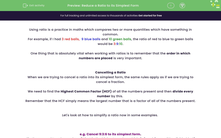# Reduce a Ratio to its Simplest Form

In this worksheet, students will practise converting values into the same units and finding the HCF to simplify ratios with three or more elements into their simplest possible form.Key stage:  KS 4

Year:  GCSE

GCSE Subjects:   Maths

GCSE Boards:   AQA, Eduqas, Pearson Edexcel, OCR,

Curriculum topic:   Ratio, Proportion and Rates of Change

Curriculum subtopic:   Ratio, Proportion and Rates of Change Calculations with Ratio

Difficulty level:#### Worksheet Overview

Using ratio is a practice in maths which compares two or more quantities which have something in common.

For example, if I had 3 red balls,  9 blue balls and 10 green balls, the ratio of red to blue to green balls would be 3:9:10.

One thing that is absolutely vital when working with ratios is to remember that the order in which numbers are placed is very important.

Cancelling a Ratio

When we are trying to cancel a ratio into its simplest form, the same rules apply as if we are trying to cancel a fraction.

We need to find the Highest Common Factor (HCF) of all the numbers present and then divide every number by this.

Remember that the HCF simply means the largest number that is a factor of all of the numbers present.

Let's look at how to simplify a ratio now in some examples.

e.g. Cancel 9:3:6 to its simplest form.

Firstly, we need to ask ourselves: "What is the highest number which 9, 3 and 6 can all be divided by?"

The HCF of 9,3 and 6 is 3.

Now we need to divide every section of the ratio by this number:

9 ÷ 3 = 3

3 ÷ 3 = 1

6 ÷ 3 = 2

So 9:3:6 can be simplified to 3:1:2.

e.g. Simplify 25:85:15.

The HCF of 25, 85 and 15 is 5.

Now we need to divide every section of the ratio by this number:

25 ÷ 5 = 5

85 ÷ 5 = 17

15 ÷ 5 = 3

So 25:85:15 can be simplified to 5:17:3.

e.g. Write £2 to 30 p to 45 p as a ratio in its simplest form.

The key thing to notice here is that the units aren't the same so we need to convert these to the same format before we start.

There is 100p in £1, so £2 = 200 p.

Remember, as well, that the order of the ratio must be the same as in the question:

£2: 30 p:45 p

Now we have created a ratio with each number in the same format, we can remove the units and start to cancel it down:

200:30:45

The HCF of 200, 30 and 45 is 5.

Now we need to divide every section of the ratio by this number:

200 ÷ 5 = 40

30 ÷ 5 = 6

45 ÷ 5 = 9

So 200:30:45 can be simplified to 40:6:9.

In this activity, we will apply the process shown above of converting values into the same units and finding the HCF to simplify ratios with three or more elements into their simplest possible form.

### What is EdPlace?

We're your National Curriculum aligned online education content provider helping each child succeed in English, maths and science from year 1 to GCSE. With an EdPlace account you’ll be able to track and measure progress, helping each child achieve their best. We build confidence and attainment by personalising each child’s learning at a level that suits them.

Get started